### Subtracting Fractions − When the denominator is different

• #### Example 1.      1/2 − 1/4 =

•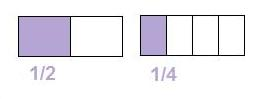(a) Multiply the top and bottom of the first fraction by the second denominator.
(b) Multiply the top and bottom of the second fraction by the first denominator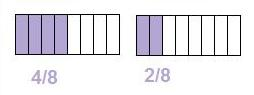(c) subtract the numerators

= 2/8(d) write fraction in its simplest form

= 1/4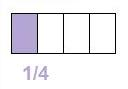• #### Example 2.      5/6 − 2/3 =

•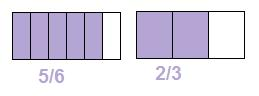(a) Multiply the top and bottom of the first fraction by the second denominator.
(b) Multiply the top and bottom of the second fraction by the first denominator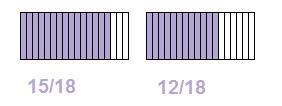(c) subtract the numerators

= 3/18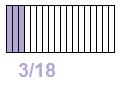(d) write fraction in its simplest form

= 1/6to: